Open in App
Not now

# Class 12 RD Sharma Solutions – Chapter 21 Areas of Bounded Regions – Exercise 21.1 | Set 3

• Last Updated : 20 May, 2021

### Question 21. Draw a rough sketch of the curveand find the area between x-axis, the curve and the ordinates x = 0, x = π

Solution:

Here, we have to find the bounded byx-axis, x = 0 and x = π

Here is the table for values ofHere is the rough sketch,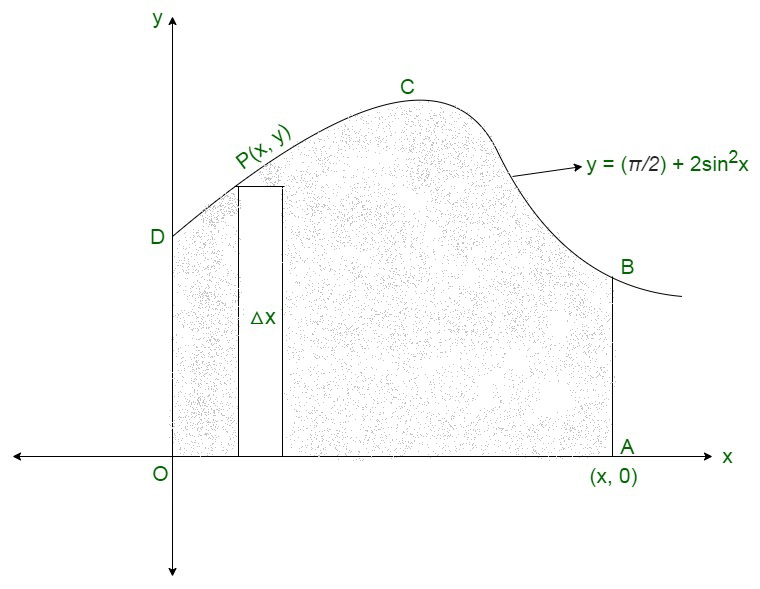Shaded region represents the required area.

We slice it into approximation rectangle of

Width = △x

Length = y

Area of rectangle = y△x

The approx rectangles slide from x = 0 to x = π,

Thus,

Required area = Region ABCDORequired area =square units

### Question 22. Draw a rough sketch of the curveand find the area between the x-axis, the curve and the ordinates x = 0, x = π.

Solution:

Here, we have the area between y-axis,

x = 0,

x = π

andThus, the table for equation (1) is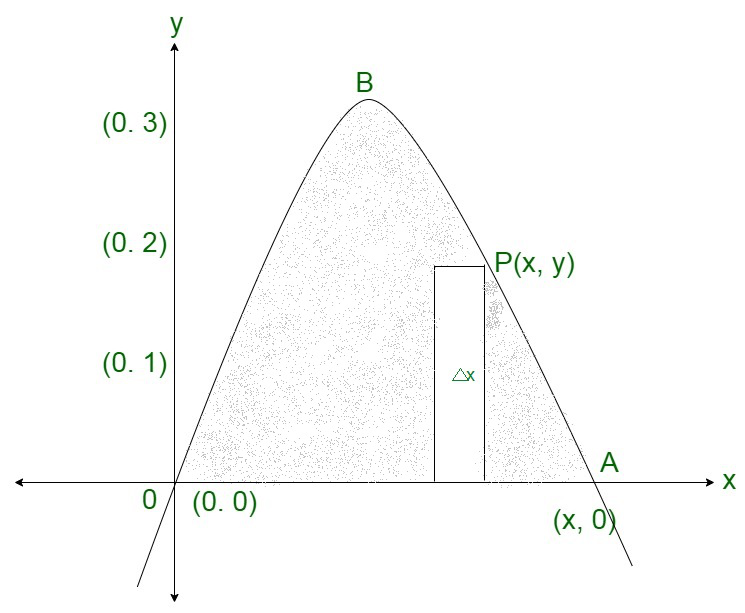Shaded region represents the required area.

We slice it into approximation rectangle of

Width = △x

Length = y

Area of rectangle = y△x

The approx rectangles slide from x = 0 to x = π,

Thus,

Required area = Region ABOARequired area =square units

### Question 23. Find the area bounded by the curve y = cos x between x = 0 and x = 2π

Solution: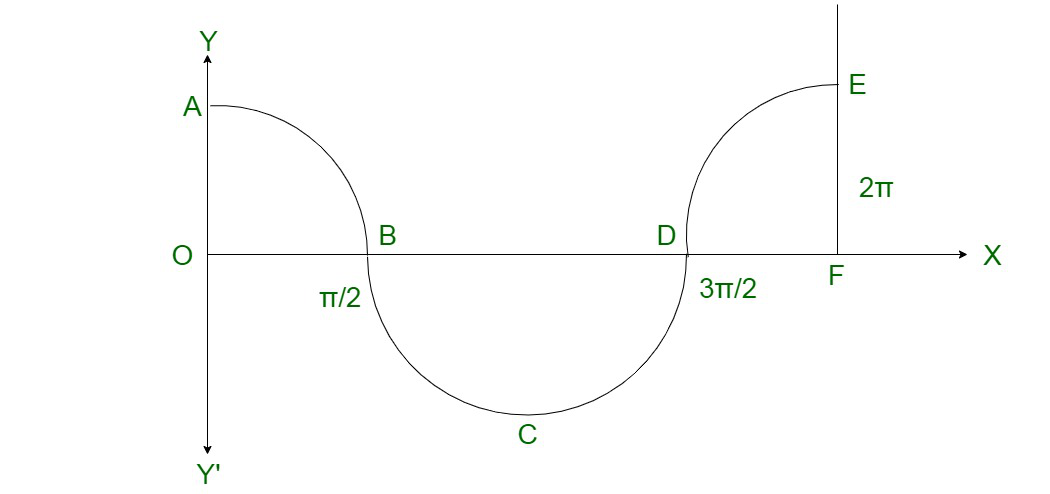Here from the figure we can see that

The required area = area of the region OABO + area of the region BCDB + area of the region DEFD

Therefore,

The required area =### Question 24. Show that the areas under the curves y = sin x and y = sin 2x between x = 0 and x =are the ratio 2:3.

Solution:

We have to find the area under the curve

y = sin x    ……..(1)

and

y = sin 2x     …………(2)

Between x = 0 and x =Here is the rough sketch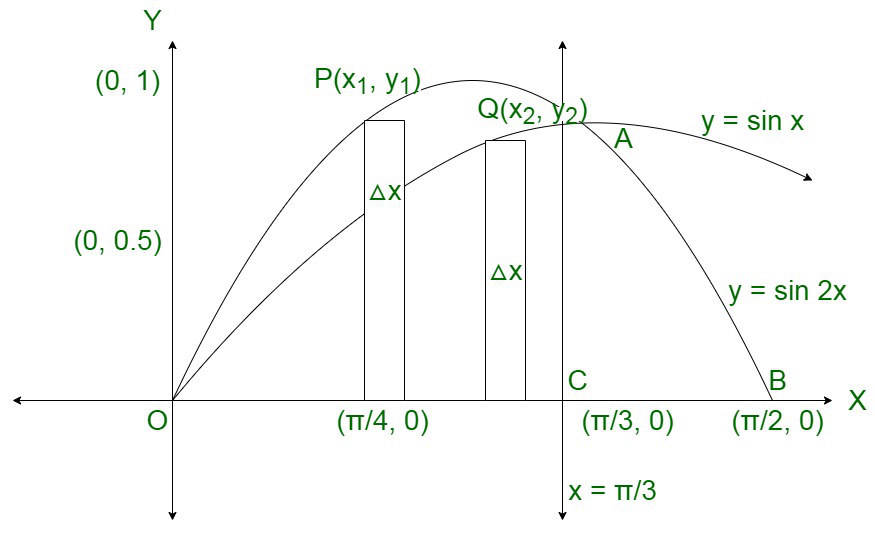Area under curve y = sin 2x

Shaded region represents the required area.

We slice it into approximation rectangle of

Width = △x

Length = y1

Area of rectangle = y1△x

The approx rectangles slide from x = 0 to x =,

Thus,

Required area = Region OPACOWe slice it into approximation rectangle of

Width = △x

Length = y2

Area of rectangle = y2△x

The approx rectangles slide from x = 0 to x =,

Thus,

Required area = Region OQACOThus,### Question 25. Compare the area under the curves y = cos2x and y = sin2x between x = 0 and x = π

Solution:

Here to compare area under curves

y = cos2x

and

y = sin2x

Between x = 0 and x = π

This is the table for y = cos2x and y = sin2x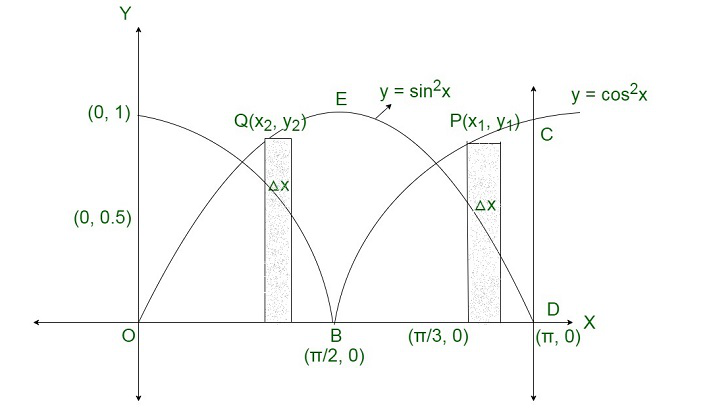Area of region enclosed by

y = cos2x and axis

A1 = Region OABO + Region BCDB

= 2(Region BCDB)Area of region enclosed by y = sin2x and axis

A2 = Region OEDOFrom equation (1) and (2),

A1 = A2

Thus,

Area enclosed by y = cos2x = Area enclosed by y = sin2x.

### Question 26. Find the area bounded by the ellipseand the ordinates x = 0 and x = ae, where, b2 = a2(1 – e 2) and e < 1.

Solution:

Thus, the required area in the figure below of the region BOB’RFSB is enclosed by the ellipse and the lines x = 0 and x = ae

Here is the area of the region BOB’RFSB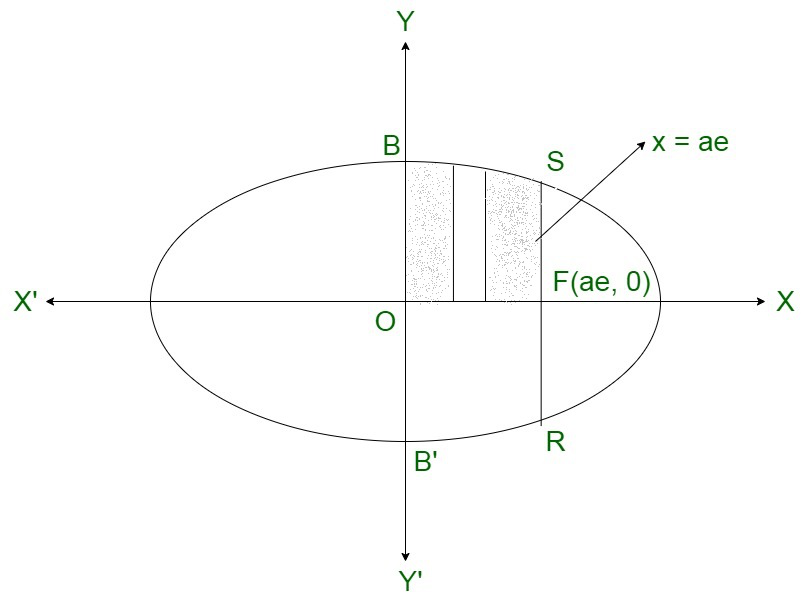### Question 27. Find the area of the minor segment of the circle x2 + y2 = a2 cut-off by the line x =.

Solution: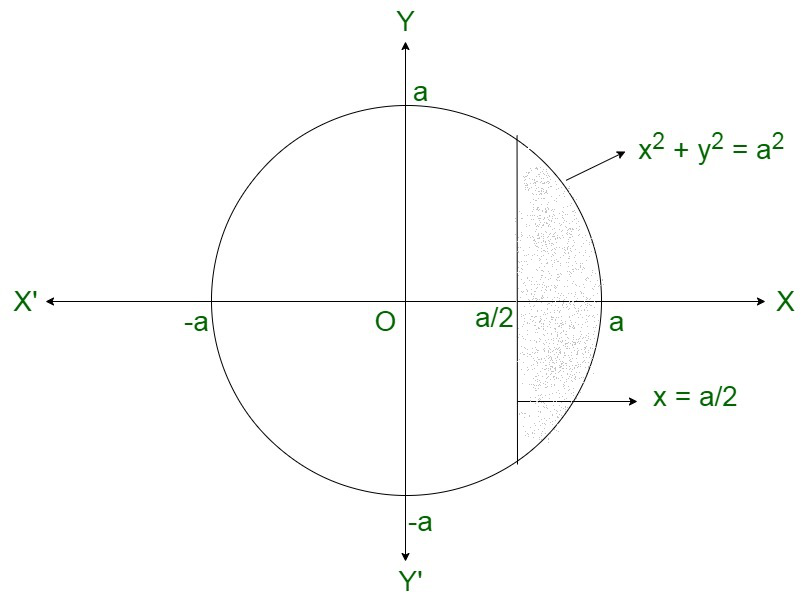Area of the mirror segment of the circle### Question 28. Find the area of the region bounded by the curve x = at, y = 2at between the ordinates corresponding t = 1 and t = 2.

Solution: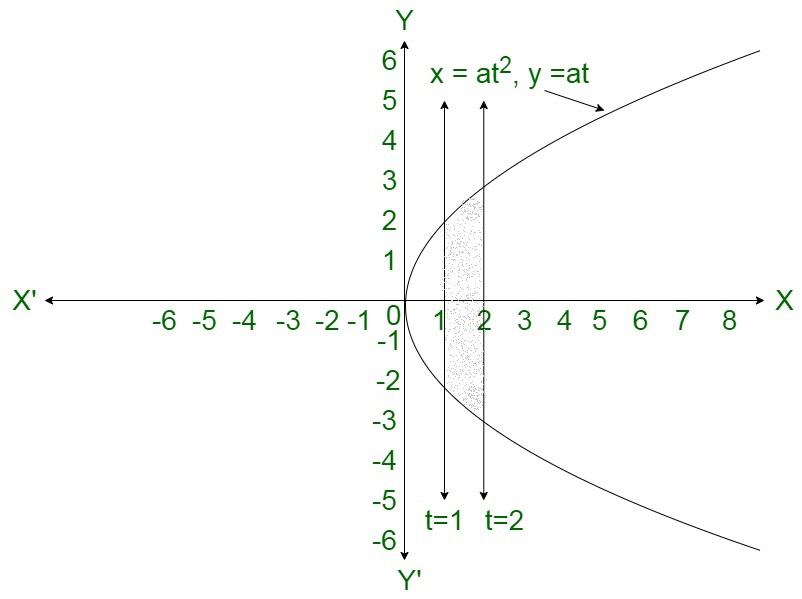Area of the bounded region### Question 29. Find the area enclosed by the curve x = 3 cos t, y = 2 sin t.

Solution: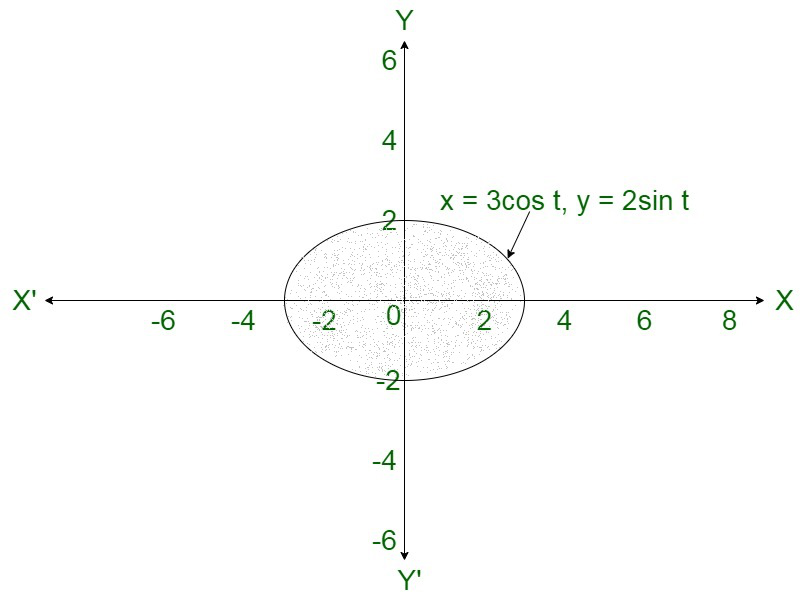Area of the bounded region= -8 [0 – 1]

= 8 square units

My Personal Notes arrow_drop_up